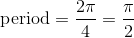## Example Questions

### Example Question #53 : Trigonometry

What is the period of 2sin(4Θ)?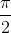None of the answers are correctExplanation:

The period of sinΘ is 2Π, so we set the new angle equal to the base period of 2Π and solve for Θ.

So 4Θ = 2Π and Θ = Π/2.

### Example Question #54 : Trigonometry

A function with period P will repeat on intervals of length P, and these intervals are referred to as periods.

Find the period of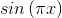.Explanation:

For the function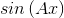the period is equal to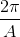in this case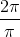which reduces to.

### Example Question #55 : Trigonometry

A function with period P will repeat on intervals of length P, and these intervals are referred to as periods.

Find the period of the function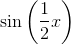.Explanation:

For the functionthe period is equal toin this case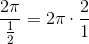which reduces to.

### Example Question #56 : Trigonometry

What is the period of the function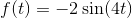?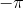Explanation:

To find the period of Sine and Cosine functions you use the formula: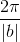wherecomes from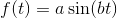. Looking at our formula you see b is 4 so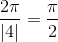### Example Question #2971 : Act Math

What is the period of the given trigonometric function: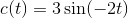. Leave your answer in terms of, simplify all fractions.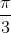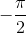Explanation:

To find the period of a sine, cosine, cosecant, or secant funciton use the formula: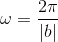wherecomes from the general formula: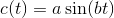. We see that for our equation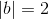and so the period iswhen you reduce the fraction.

### Example Question #1 : How To Find The Period Of The Sine

Find the period of the following formula: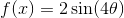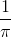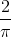Explanation:

To find period, simply remember the following formula: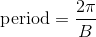where B is the coefficient in front of x. Thus,